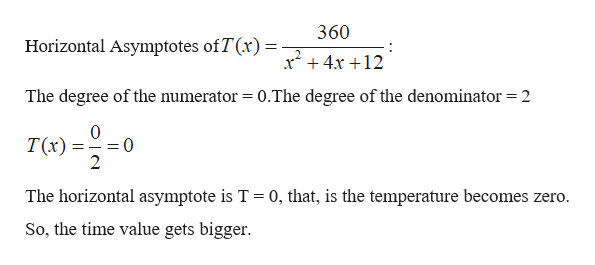# Suppose than an object that is originally at room temperature of 30 Celsius is placed in a freezer. The temperature T (x) of the object can be approximated by the model T (x) = 360 / xˆ2 + 4x + 12, where x is the time in hours after the object is placed in the freezer.a) What is the horizontal asymptote of the graph of this function and what does it represent in the context of this problem?b) A chemist needs a compound cooled to less than 5 Celsius. Determine the amount of time required for the compound to cool so that its temperature is less than 5 Celsius. PLEASE DO NOT USE LIMIT TO SOLVE IT.......I CAN NOT USE LIMIT IN THIS CLASS.

Question
45 views

Suppose than an object that is originally at room temperature of 30 Celsius is placed in a freezer. The temperature T (x) of the object can be approximated by the model T (x) = 360 / xˆ2 + 4x + 12, where x is the time in hours after the object is placed in the freezer.

a) What is the horizontal asymptote of the graph of this function and what does it represent in the context of this problem?

b) A chemist needs a compound cooled to less than 5 Celsius. Determine the amount of time required for the compound to cool so that its temperature is less than 5 Celsius.

PLEASE DO NOT USE LIMIT TO SOLVE IT.......I CAN NOT USE LIMIT IN THIS CLASS.

check_circle

Step 1

Part a)

Given,

Step 2

To find the horizontal asymptotes of the given function:

If denominator’s degree >numer...help_outlineImage Transcriptionclose360 Horizontal Asymptotes ofT(x) 2 x4x12 The degree of the numerator 0.The degree of the denominator=2 0 T(x) 2 The horizontal asymptote is T 0, that, is the temperature becomes zero. So, the time value gets bigger fullscreen

### Want to see the full answer?

See Solution

#### Want to see this answer and more?

Solutions are written by subject experts who are available 24/7. Questions are typically answered within 1 hour.*

See Solution
*Response times may vary by subject and question.
Tagged in

### Algebra